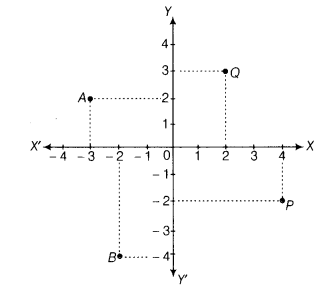# Look at the given figure and answer the following questions

Look at the given figure and answer the following questions(i) What is the abscissa of P?
(ii) What is the ordinate of Q?
(iii) What are the coordinates of origin?
(iv) Which point is having abscissa as (-3)?

(i) The abscissa of P is 4.
(ii) The ordinate of Q is 3.
(iii) The coordinates of origin are (0, 0).
(iv) The point A is having abscissa as (-3).